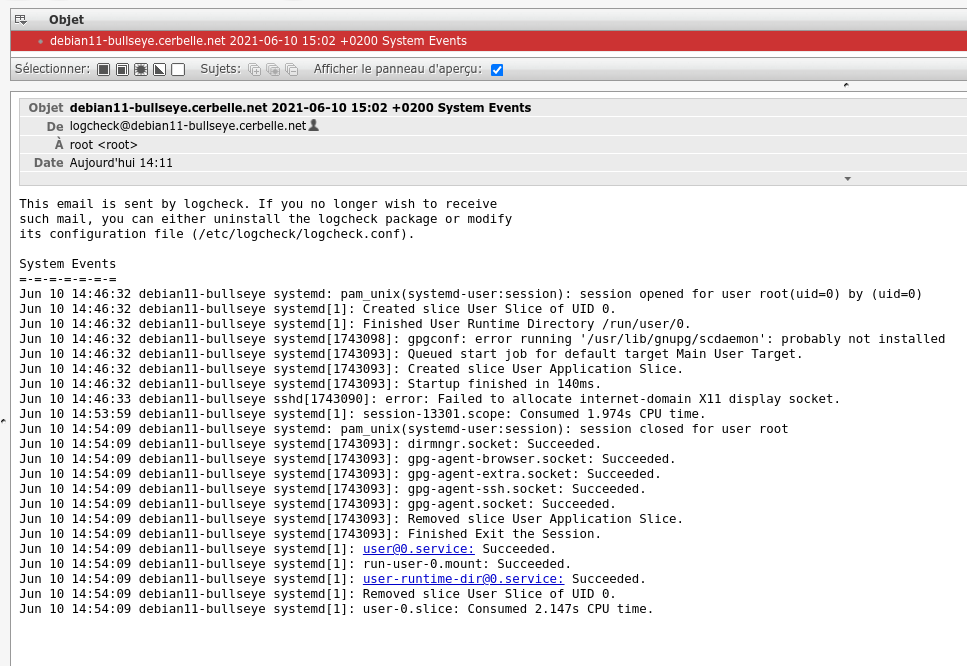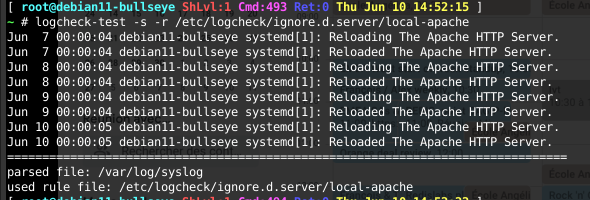Logcheck installation and configuration with tuning. Logcheck parses the system logfiles, removing known legitimate patterns and sends the remaining lines to the administrator. It reports all the unusual activity, helping to detect attack attempts or successful attacks that would not be catched by other tools. I prefer to have less notifications, and to read them all, instead of having too many and skip them.# Logcheck presentation

LogCheck is a part of the global security of the servers. Basically, it parses all the main log files up-to-now, removing the known standard usual patterns and it sends the remaining lines to the administrator. It stores a cursor per log file to avoid parsing several times the same lines.

My policy is to consider the already blocked attacks as normal, because they were blocked and might be further blocked later, automatically. I am only interested in the unknown patterns. It would be useless to have so many notifications that I would not read them. I prefer to have less, and to read them. On my test system, in a protected network, my goal is to have no notification at all. If I receive a notification everyday when cron-daily triggers, I do not read it anymore and if there is an attack in this timeslot, I’ll miss it.

# Prerequisites

## Existing variables

We need the `LAN_*` and `WAN_*` variables which were already defined in the configuration file, the Configuration variables and the Network configuration posts.

Ensure that the variables are available, by loading the configuration script :

``````source /root/config.env
``````

# Installation

Let’s install the tool with `apt-get`.

``````apt-get install -y logcheck
``````

# Configuration and tuning

## Aggregate (group/count) log lines

First, I do not want an extensive list of the remaining log lines in the notification emails, I prefer to have the similar lines grouped together, the email is more compact and as useful. The idea is to get notified and to potentially investigate further on the server itself, not to store logs in my mailbox. Then, I also configure the email address to send the notifications to.

``````sed -i 's/^#\?SYSLOGSUMMARY=0/SYSLOGSUMMARY=1/' /etc/logcheck/logcheck.conf
sed -i 's/^#\?\(SENDMAILTO=\).*/\1"root"/' /etc/logcheck/logcheck.conf
``````

## Useful filtering patterns

• Interface `en[op][0-9]s[0-9](p[0-9])?`
• IP4 `([0-9]{1,3}\.){3}[0-9]{1,3}`
• IP6 `([[:xdigit:]]{4}:){7}[[:xdigit:]]{4}`
• MAC `([[:xdigit:]]{2}:){13}[[:xdigit:]]{2}`

I strongly advice to always wrap the regular expressions between a carret and a dollar sign, to represent the whole line and avoid missing a useful line.

## Ignore IPTables blocked attacks

IPTables still logs a lot of blocked connections. I don’t want to be notified about the blocked connections, they were blocked. I want to be notified about unusual activity, thus I filter all the already detected and managed issues. I keep only attemps LOG/DROPed by the generic catchall rule. These rules are very specific and restrictive to really known patterns, thus, it can leave a lot of remaining lines. If needed, I can adjust them to be less restrictive.

``````cat << EOF > /etc/logcheck/ignore.d.server/local-kernel
^\w{3} [ :[:digit:]]{11} [-._[:alnum:]]+ kernel: \[[ [:digit:]]+\.[[:digit:]]+\] \[STEALTH\] IN=[[:alnum:]]+ OUT= MAC=[[:alnum:]:]+ SRC=[.[:digit:]]{7,15} DST=[.[:digit:]]{7,15} LEN=[[:digit:]]+ TOS=0x[[:digit:]]+ PREC=0x[[:digit:]]+ TTL=[[:digit:]]+ ID=[[:digit:]]+ .*\$
^\w{3} [ :[:digit:]]{11} [-._[:alnum:]]+ kernel: \[[ [:digit:]]+\.[[:digit:]]+\] \[SYNSCAN\] IN=[[:alnum:]]+ OUT= MAC=[[:alnum:]:]+ SRC=[.[:digit:]]{7,15} DST=[.[:digit:]]{7,15} LEN=[[:digit:]]+ TOS=0x[[:digit:]]+ PREC=0x[[:digit:]]+ TTL=[[:digit:]]+ ID=[[:digit:]]+ .*\$
^\w{3} [ :[:digit:]]{11} [-._[:alnum:]]+ kernel: \[[ [:digit:]]+\.[[:digit:]]+\] \[CNSCAN\] IN=[[:alnum:]]+ OUT= MAC=[[:alnum:]:]+ SRC=[.[:digit:]]{7,15} DST=[.[:digit:]]{7,15} LEN=[[:digit:]]+ TOS=0x[[:digit:]]+ PREC=0x[[:digit:]]+ TTL=[[:digit:]]+ ID=[[:digit:]]+ .*\$
^\w{3} [ :[:digit:]]{11} [-._[:alnum:]]+ kernel: \[[ [:digit:]]+\.[[:digit:]]+\] \[GRSCAN\] IN=[[:alnum:]]+ OUT= MAC=[[:alnum:]:]+ SRC=[.[:digit:]]{7,15} DST=[.[:digit:]]{7,15} LEN=[[:digit:]]+ TOS=0x[[:digit:]]+ PREC=0x[[:digit:]]+ TTL=[[:digit:]]+ ID=[[:digit:]]+ .*\$
^\w{3} [ :[:digit:]]{11} [-._[:alnum:]]+ kernel: \[[ [:digit:]]+\.[[:digit:]]+\] \[INPUT\] IN=\${WAN_IF} OUT= MAC=([[:xdigit:]]{2}:){13}[[:xdigit:]]{2} SRC=([0-9]{1,3}\.){3}[0-9]{1,3} DST=([0-9]{1,3}\.){3}255 LEN=164 TOS=0x00 PREC=0x00 TTL=64 ID=[[:digit:]]+ DF PROTO=UDP SPT=44752 DPT=6771 LEN=[[:digit:]]+\$
^\w{3} [ :[:digit:]]{11} [-._[:alnum:]]+ kernel: \[[ [:digit:]]+\.[[:digit:]]+\] \[INPUT\] IN=\${WAN_IF} OUT= MAC=([[:xdigit:]]{2}:){13}[[:xdigit:]]{2} SRC=([0-9]{1,3}\.){3}[0-9]{1,3} DST=([0-9]{1,3}\.){3}255 LEN=44 TOS=0x00 PREC=0x00 TTL=64 ID=[[:digit:]]+ DF PROTO=UDP SPT=8612 DPT=861 LEN=24\$
^\w{3} [ :[:digit:]]{11} [-._[:alnum:]]+ kernel: \[[ [:digit:]]+\.[[:digit:]]+\] \[INPUT\] IN=\${WAN_IF} OUT= MAC=([[:xdigit:]]{2}:){13}[[:xdigit:]]{2} SRC=([0-9]{1,3}\.){3}[0-9]{1,3} DST=255\.255\.255\.255 LEN=101 TOS=0x00 PREC=0x00 TTL=64 ID=[[:digit:]]+ DF PROTO=UDP SPT=[[:digit:]]+ DPT=161 LEN=81\$
^\w{3} [ :[:digit:]]{11} [-._[:alnum:]]+ kernel: \[[ [:digit:]]+\.[[:digit:]]+\] \[INPUT\] IN=\${WAN_IF} OUT= MAC=([[:xdigit:]]{2}:){13}[[:xdigit:]]{2} SRC=([0-9]{1,3}\.){3}[0-9]{1,3} DST=255.255.255.255 LEN=[[:digit:]]+ TOS=0x0 PREC=0x00 TTL=[[:digit:]]+ ID=[[:digit:]]+ (DF )?PROTO=UDP SPT=68 DPT=67 LEN=[[:digit:]]+\$
# ff02:0000:0000:0000:0000:0000:0000:0002 = All local routers
# FF02:0000:0000:0000:0000:0000:0000:0001 = All local nodes
^\w{3} [ :[:digit:]]{11} [-._[:alnum:]]+ kernel: \[[ [:digit:]]+\.[[:digit:]]+\] \[IP6\] IN= OUT=en[op][0-9]s[0-9](p[0-9])? SRC=([[:xdigit:]]{4}:){7}[[:xdigit:]]{4} DST=ff02:(0000:){6}0002 LEN=56 TC=0 HOPLIMIT=255 FLOWLBL=0 PROTO=ICMPv6 TYPE=133 CODE=0\$
^\w{3} [ :[:digit:]]{11} [-._[:alnum:]]+ kernel: \[[ [:digit:]]+\.[[:digit:]]+\] \[IP6\] IN= OUT=en[op][0-9]s[0-9](p[0-9])? SRC=(0000:){7}0000 DST=ff02:(0000:){6}0016 LEN=76 TC=0 HOPLIMIT=1 FLOWLBL=0 PROTO=ICMPv6 TYPE=143 CODE=0 MARK=0xd4\$
^\w{3} [ :[:digit:]]{11} [-._[:alnum:]]+ kernel: \[[ [:digit:]]+\.[[:digit:]]+\] \[IP6\] IN= OUT=lo SRC=(0000:){7}0001 DST=(0000:){7}0001 LEN=[[:digit:]]+ TC=[[:digit:]]+ HOPLIMIT=64 FLOWLBL=[[:digit:]]+ PROTO=UDP SPT=[[:digit:]]+ DPT=[[:digit:]]+ LEN=[[:digit:]]+\$
EOF
``````

## Ignore blocked SSH connections

These lines are typical SSH connection failures. The connections were blocked, then the SSH rate limiter and the fail2ban rules will take actions if the attack continue. I don’t need to be notified.

``````cat << EOF > /etc/logcheck/ignore.d.server/local-ssh
^\w{3} [ :[:digit:]]{11} [-._[:alnum:]]+ sshd\[[[:digit:]]+\]: Received disconnect from [[:digit:].]+ port [[:digit:]]+.*\[preauth\]\$
^\w{3} [ :[:digit:]]{11} [-._[:alnum:]]+ sshd\[[[:digit:]]+\]: Disconnected from [[:digit:].]+ port [[:digit:]]+.*\[preauth\]\$
^\w{3} [ :[:digit:]]{11} [-._[:alnum:]]+ sshd\[[[:digit:]]+\]: Invalid user [_[:alnum:]]+ from [[:digit:].]+ port [[:digit:]]+\$
^\w{3} [ :[:digit:]]{11} [-._[:alnum:]]+ sshd\[[[:digit:]]+\]: Connection closed by [[:digit:].]+ port [[:digit:]:]+.*\[preauth\]\$
EOF
``````

## Systemd normal activity

These are normal maintenance actions automatically triggered by `systemd`. I don’t care when it works ! ;)

``````cat << EOF > /etc/logcheck/ignore.d.server/local-systemd
^\w{3} [ :[:digit:]]{11} [-._[:alnum:]]+ systemd\[1\]: [-/:. [:alnum:]]+: Succeeded.\$
^\w{3} [ :[:digit:]]{11} [-._[:alnum:]]+ systemd\[1\]: [-/:. [:alnum:]]+: Succeeded.\$
^\w{3} [ :[:digit:]]{11} [-._[:alnum:]]+ systemd\[1\]: Started [-/:. [:alnum:]]+\$
^\w{3} [ :[:digit:]]{11} [-._[:alnum:]]+ systemd\[1\]: Created [-/:. [:alnum:]]+\$
^\w{3} [ :[:digit:]]{11} [-._[:alnum:]]+ systemd\[1\]: Starting [-/:. [:alnum:]]+\$
^\w{3} [ :[:digit:]]{11} [-._[:alnum:]]+ systemd\[1\]: Finished [-/:. [:alnum:]]+\$
EOF
``````

## CronApt

CronApt tells us that it managed to update its package database and to send us a notification… I don’t need a double notification.

``````cat << EOF > /etc/logcheck/ignore.d.server/local-cronapt
^\w{3} [ :[:digit:]]{11} [-._[:alnum:]]+ cron-apt: CRON-APT ACTION: 9-notify\$
^\w{3} [ :[:digit:]]{11} [-._[:alnum:]]+ cron-apt: CRON-APT LINE: /usr/bin/apt-get -o quiet=2 -q -q --no-act upgrade\$
^\w{3} [ :[:digit:]]{11} [-._[:alnum:]]+ cron-apt: CRON-APT LINE: /usr/bin/apt-get -o quiet=2 update -o quiet=2\$
EOF
``````

## Miscellaneous

There is an audit procedure regularly triggered in the linux kernel to gather some metrics about performances. I has to be as transparent as possible, its trigger period is increased if the call takes too long. This is somehow a normal message, at least until the ideal period is found. Anyway, I would have other visible issues before this message could be useful. I also grouped here some log lines about fail2ban, dhcp, ntpd, RKHunter and rsyslogd normal behavior. RKHunter already sends emails by itself, no need to duplicate.

``````cat << EOF > /etc/logcheck/ignore.d.server/local-misc
^\w{3} [ :[:digit:]]{11} [-._[:alnum:]]+ kernel: \[[ [:digit:]]+\.[[:digit:]]+\] perf: interrupt took too long \([[:digit:]]+ > [[:digit:]]+\), lowering kernel.perf_event_max_sample_rate to [[:digit:]]+\$
^\w{3} [ :[:digit:]]{11} [-._[:alnum:]]+ fail2ban-server\[[[:digit:]]+\]: Server ready\$
^\w{3} [ :[:digit:]]{11} [-._[:alnum:]]+ isc-dhcp-server\[[[:digit:]]+\]: Starting ISC DHCPv4 server: dhcpd.\$
^\w{3} [ :[:digit:]]{11} [-._[:alnum:]]+ ntpd\[[[:digit:]]+\]: configuration OK\$
^\w{3} [ :[:digit:]]{11} [-._[:alnum:]]+ rsyslogd:  \[origin software="rsyslogd" swVersion="8.1901.0" x-pid="[[:digit:]]+" x-info="https://www.rsyslog.com"\] rsyslogd was HUPed\$
^\w{3} [ :[:digit:]]{11} [-._[:alnum:]]+ rkhunter: Please inspect this machine, because it may be infected.\$
EOF
``````

## Second NIC specific rules

If there is a second NIC on the server, and if it is used as a router, the internal LAN can be restarted, unplugged, … Thus, I don’t want to be notified by email, it would be stupid to send the notification somewhere, in a location that I can not reach ;) Furthermore, these rules are specific to my hardware (NIC and Switch).

``````cat << EOF > /etc/logcheck/ignore.d.server/local-router
^\w{3} [ :[:digit:]]{11} [-._[:alnum:]]+ kernel: \[\s?[[:digit:]]+\.[[:digit:]]+\] e1000e 0000:03:00.0 enp3s0: 10/100 speed: disabling TSO\$
^\w{3} [ :[:digit:]]{11} [-._[:alnum:]]+ kernel: \[\s?[[:digit:]]+\.[[:digit:]]+\] e1000e: \${LAN_IF} NIC Link is Down\$
^\w{3} [ :[:digit:]]{11} [-._[:alnum:]]+ kernel: \[\s?[[:digit:]]+\.[[:digit:]]+\] e1000e: \${LAN_IF} NIC Link is Up 1000 Mbps Full Duplex, Flow Control: Rx/Tx\$
``````

# Tests

## Logcheck-test

If you are unsure about rules, you can use the `logcheck-test` tool to apply a rule file on a logfile, either to get the matches or to get the remaining lines. This is not part of my standard installation, but I wanted to note this information here as it could be useful to debug rules and RegExps.## Rules test

This test will execute logcheck with a real configuration, but will not update the log cursor, thus you can execute it as many times as you want. It does not send a notification email neither.

``````sudo -u logcheck logcheck -o -t
``````

``````sudo -u logcheck logcheck -t